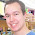## Pages

### XNA Sphere

As mentioned in the previous post, this is bout creating a sphere in XNA.
I forgot to mention in my previous post, that these are useful for visualizing stuff in 3d world, this actually doesn't create surface - just lines.

I'll be using the Circle created in before to setup my sphere.

The funny thing about a sphere is that projection on any plane will always be a circle.Hence, when creating the circles we ensure that they lie on the circumference

Refer image below for clarity:

Here is the complete code:

`//Generic Sphere for XNA Debugging / Visualizing.`
`//This is just a set of lines - no surface`
`//Author: Vikram N [ makubex ] `
`//Blog: 8bitmemories.blogspot.com`
`public class Sphere`
`{        `
`//Color of the verttices`
`Color _VertexColor;`
` `
`//List of Circles that make up the sphere`
`//Refer to post on Circles`
`List<Circle> _Verticals;`
`List<Circle> _Horizontals;`
` `
`//Save original position`
`//Becomes easy to transform the sphere`
`Vector3[] _HBasePositions;`
`Vector3[] _VBasePositions;`
` `
`//Main game reference`
`Game _Game;`
`BasicEffect _Effect;`
` `
`//Not used`
`//NFS: May be useful someother day`
`Matrix _Transforms;`
`//Store pos, scale and rotation`
`//Only pos is useful. Others have not been implemented`
`//Rotation - no diff`
`//Scale - why dude..  create a new one with diff radius :P`
`Vector3 _Position, _Scale, _Rotation;`
`//Radius of the sphere`
`float _Radius;`
` `
`public Vector3 Position`
`{`
`get`
`{`
`return _Position;`
`}`
` `
`set`
`{`
`_Position = value;`
`Transform();`
`}`
`}`
` `
`public Vector3 Rotation`
`{`
`get`
`{`
`return _Rotation;`
`}`
` `
`set`
`{`
`_Rotation = value;`
`Transform();`
`}`
`}`
` `
`public Vector3 Scale`
`{`
`get`
`{`
`return _Scale;`
`}`
` `
`set`
`{`
`_Scale = value;`
`Transform();`
`}`
`}`
` `
`public Matrix Transforms`
`{`
`get`
`{`
`return _Transforms;`
`}`
` `
`set`
`{`
`_Transforms = value;`
`}`
`}`
` `
`//No of circles to be used for creating the sphere`
`//Higher the number, better it looks`
`//10 seems to look nice :P`
`int LEVELS = 10;`
`//Constructor`
`public Sphere(Game game, Color color, float radius)`
`{`
`_Game = game;`
`_Effect = new BasicEffect(_Game.GraphicsDevice, null);`
`_Effect.VertexColorEnabled = true;`
`_VertexColor = color;`
`_Transforms = Matrix.Identity;`
`_Radius = radius;`
` `
`_Verticals = new List<Circle>();`
`_Horizontals = new List<Circle>();`
` `
`Setup();`
`}`
` `
`//Function to create circles`
`void Setup()`
`{`
`float offset = _Radius / (float)LEVELS;`
`float rOffset = 90 / LEVELS;`
`//Add one on each side of the center`
`//For expln refer the blog - 8bitmemories.blogspot.com`
`for (int i = 0; i <= LEVELS; i++)`
`{        `
`Circle tmpPos = new Circle(_Game, _VertexColor, _Radius * (float) Math.Cos(MathHelper.ToRadians(i*rOffset)));`
`tmpPos.Position = new Vector3(0, _Radius * (float)Math.Sin(MathHelper.ToRadians(i * rOffset)), 0);`
` `
`Circle tmpNeg = new Circle(_Game, _VertexColor, _Radius * (float)Math.Cos(MathHelper.ToRadians(i * rOffset)));`
`tmpNeg.Position = new Vector3(0, -_Radius * (float)Math.Sin(MathHelper.ToRadians(i * rOffset)), 0);`
` `
`_Verticals.Add(tmpPos);`
`_Verticals.Add(tmpNeg);`
`}`
` `
`//Same logic but set rotation of 90 degrees on z axis`
`for (int i = 0; i <= LEVELS; i++)`
`{`
`Circle tmpPos = new Circle(_Game, _VertexColor, _Radius * (float)Math.Cos(MathHelper.ToRadians(i * rOffset)));`
`tmpPos.Position = new Vector3(_Radius * (float)Math.Sin(MathHelper.ToRadians(i * rOffset)), 0, 0);`
`tmpPos.Rotation = new Vector3(0, 0, MathHelper.PiOver2);`
` `
`Circle tmpNeg = new Circle(_Game, _VertexColor, _Radius * (float)Math.Cos(MathHelper.ToRadians(i * rOffset)));`
`tmpNeg.Position = new Vector3( -_Radius * (float)Math.Sin(MathHelper.ToRadians(i * rOffset)),0,  0);`
`tmpNeg .Rotation = new Vector3(0, 0, MathHelper.PiOver2);`
` `
`_Horizontals.Add(tmpPos);`
`_Horizontals.Add(tmpNeg);`
`}`
` `
`//Save original positions `
`//Useful for translation        `
`_HBasePositions = _Horizontals.Select(x => x.Position).ToArray();`
`_VBasePositions = _Verticals.Select(x => x.Position).ToArray();`
` `
`}`
` `
`//Move the sphere`
`void Transform()`
`{`
`//Not used `
`_Transforms = Matrix.CreateScale(_Scale) * Matrix.CreateFromYawPitchRoll(_Rotation.Y, _Rotation.X, _Rotation.Z) * Matrix.CreateTranslation(_Position);`
` `
`//Use the base positions + translation value`
`for (int i = 0; i < _Verticals.Count; i++)`
`{`
`_Verticals[i].Position = _VBasePositions[i] + _Position;`
`}`
` `
`for (int i = 0; i < _Horizontals.Count; i++)`
`{`
`_Horizontals[i].Position = _HBasePositions[i] + _Position;`
`}`
`}`
` `
`//Draw spheres`
`public void Draw()`
`{`
`//Draw horizontal and vertical circles`
`for (int i = 0; i < _Verticals.Count; i++)`
`{`
`_Verticals[i].Draw();`
`}`
` `
`for (int i = 0; i < _Horizontals.Count; i++)`
`{`
`_Horizontals[i].Draw();`
`}`
`}`
`}`

Screen shots:

Pretty Ok for debugging I think.
NFS: I get confused with sin and cos!Hope you've learnt something in the future!

#### 3 comments:

1.FYI a full project demo included with this post would be very helpful!

2.woooow amizing
i am new at xna cant i have the project that draw

3.Can you mail me the code to soodabhi7@gmail.com ??

Note: Only a member of this blog may post a comment.